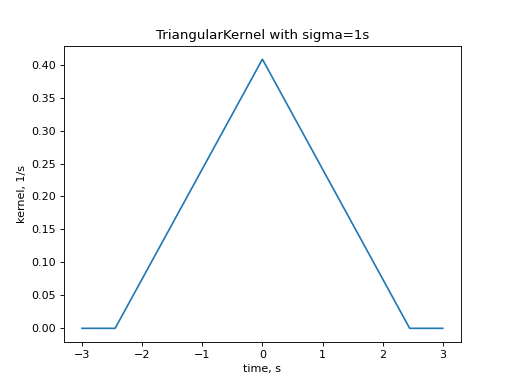elephant.kernels.TriangularKernel¶

class elephant.kernels.TriangularKernel(sigma, invert=False)[source]

Class for triangular kernels.withcorresponding to the half width of the kernel.

The parameter invert has no effect on symmetric kernels.

Examples

from elephant import kernels
import quantities as pq
import numpy as np
import matplotlib.pyplot as plt

time_array = np.linspace(-3, 3, num=1000) * pq.s
kernel = kernels.TriangularKernel(sigma=1*pq.s)
kernel_time = kernel(time_array)
plt.plot(time_array, kernel_time)
plt.title("TriangularKernel with sigma=1s")
plt.xlabel("time, s")
plt.ylabel("kernel, 1/s")
plt.show()Methods

 __init__(sigma[, invert]) boundary_enclosing_area_fraction(fraction) Calculates the boundaryso that the integral fromtoencloses a certain fraction of the integral over the complete kernel. cdf(time) Cumulative Distribution Function, CDF. icdf(fraction) Inverse Cumulative Distribution Function, ICDF, also known as a quantile. is_symmetric() True for symmetric kernels and False otherwise (asymmetric kernels). median_index(times) Estimates the index of the Median of the kernel.

Attributes

 min_cutoff Half width of the kernel.
__call__(times)

Evaluates the kernel at all points in the array times.

Parameters: timespq.QuantityA vector with time intervals on which the kernel is evaluated. pq.QuantityVector with the result of the kernel evaluations. TypeErrorIf times is not pq.Quantity. If the dimensionality of times and sigma are different.
boundary_enclosing_area_fraction(fraction)[source]

Calculates the boundaryso that the integral fromtoencloses a certain fraction of the integral over the complete kernel.

By definition the returned value is hence non-negative, even if the whole probability mass of the kernel is concentrated over negative support for inverted kernels.

Parameters: fractionfloatFraction of the whole area which has to be enclosed. pq.QuantityBoundary of the kernel containing area fraction under the kernel density. ValueErrorIf fraction was chosen too close to one, such that in combination with integral approximation errors the calculation of a boundary was not possible.
cdf(time)[source]

Cumulative Distribution Function, CDF.

Parameters: timepq.QuantityThe input time scalar. floatCDF at time.
icdf(fraction)[source]

Inverse Cumulative Distribution Function, ICDF, also known as a quantile.

Parameters: fractionfloatThe fraction of CDF to compute the quantile from. pq.QuantityThe time scalar times such that CDF(t) = fraction.
is_symmetric()

True for symmetric kernels and False otherwise (asymmetric kernels).

A kernel is symmetric if its PDF is symmetric w.r.t. time:Returns: boolWhether the kernels is symmetric or not.
median_index(times)

Estimates the index of the Median of the kernel.

We define the Median indexof a kernel as:whereandare the first and last entries of the input array, CDF and ICDF stand for Cumulative Distribution Function and its Inverse, respectively.

This function is not mandatory for symmetrical kernels but it is required when asymmetrical kernels have to be aligned at their median.

Parameters: timespq.QuantityVector with the interval on which the kernel is evaluated. intIndex of the estimated value of the kernel median. TypeErrorIf the input array is not a time pq.Quantity array. ValueErrorIf the input array is empty. If the input array is not sorted.